# Solving Differential Equations & Integrals with Technology

Instructor: Michael Eckert

Michael has a Bachelor's in Environmental Chemistry and Integrative Science. He has extensive experience in working with college academic support services as an instructor of mathematics, physics, chemistry and biology.

Solving for differential equations and integrals can often be a complex and time-consuming process. In this lesson, a scientific graphing calculator (Texas Instruments TI-84) will be used to graphically solve for both the derivative and the integral.

## Using the Texas Instruments TI-84 to Solve for Derivatives and Integrals

Sometimes, it can be a challenge and seem nearly impossible to solve for differential equations and integrals by traditional means such as identities, theorems, and algebraic manipulation. To aid us in this endeavor, we have calculators such as the Texas Instruments TI-84. In the following lesson, we will learn how to use this calculator to solve for both derivatives and integrals.Before we begin using the TI-84 to find derivatives it is best that we set both the WINDOW and MODE. MODE determines whether we can deal with decimals or just whole numbers, radians or degrees, general functions or parametric equations, and real numbers or imaginary. In this case, the MODE should be set with the following parameters: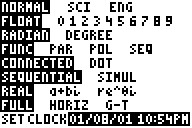We must also make sure that the WINDOW is set correctly. Before we put our function/s into the TI-84 to be graphed, we must make sure that our Cartesian coordinate system is suitable for its projection. Therefore, we set up the WINDOW as follows: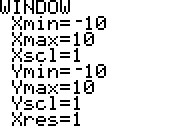Xmin = -10, Xmax = 10, Xscl = 1, Ymin = -10, Ymax = 10, Yscl = 1 and Xres = 1.

Xscl and Yscl determine how many tic-marks that we will have along the x and y-axis. Along each axis, we will have 10 in the negative direction from the origin (0,0) and 10 in the positive direction from the origin along both the x and y-axis. Xres is a variable we can just take to be 1.

## Solving for a Derivative

What if we are given y = x2 and asked to solve for dy/dx (the slope of the tangent line) at any point x?

We start by putting our function into Y = :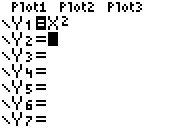After pressing the GRAPH command: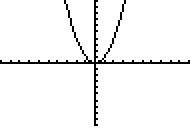We press 2nd CALC and scroll down to the derivative (dy / dx) command 6: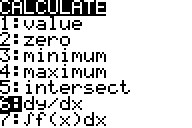We are then prompted to TRACE to any point on the graph, where we want to calculate the derivative: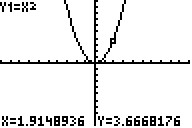After hitting the Enter, we are given the value of a derivative (the exact slope of the graph) at that exact point.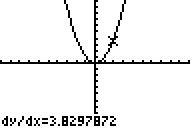## Solving for an Integral

What if we are asked to find the value of the integral (the area under the curve) from x = a to b of: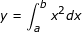To unlock this lesson you must be a Study.com Member.

### Register to view this lesson

Are you a student or a teacher?